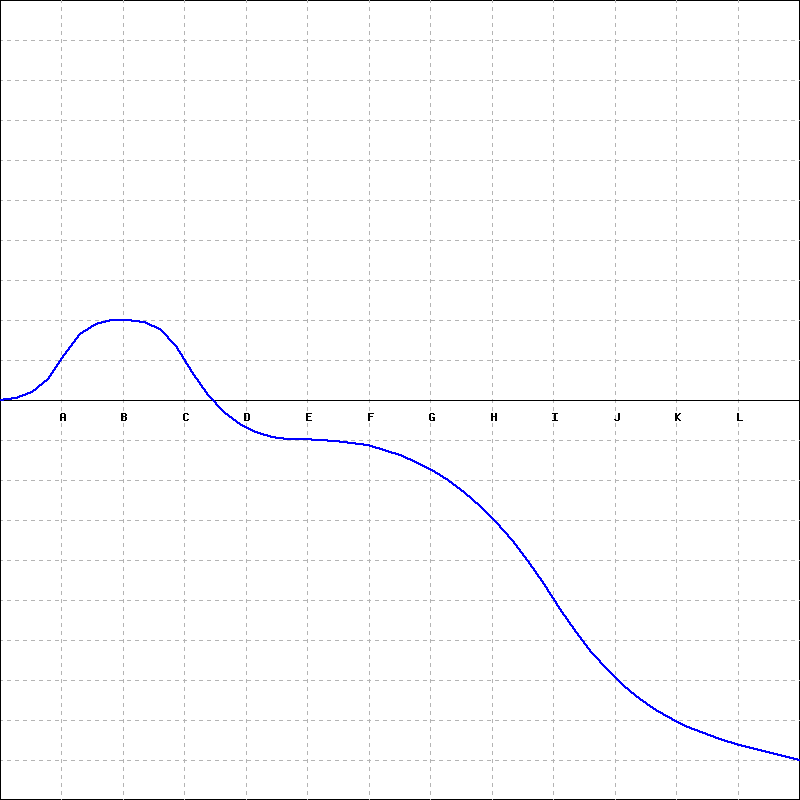# AВСDЕGНIKL

Question
3 views

How many critical points does ff have?

For each of the following questions, as your answer, list the labels of the relevant points. For instance, if a question asks you to identify the points at which the function has a local maximum, and the points at which the function has a local maximium are B, E, and H, then you should type BEH. If there are no points of the type asked for by a question, then type the word NONE .

Which points are critical points?

At which points does ff have a local maximum?

At which points does ff have a local minium?help_outlineImage TranscriptioncloseA В С D Е G Н I K L fullscreen
check_circle

Step 1

Critical points: A critical point of a continuous function is a point at which the derivative is zero or undefined.

According to the graph,

There are only 2 critical points.

One is at point B and another is at point E.

Step 2

Local maximum: The graph of a function has a local maximum at the point where the graph changes from increasing to decreasing...

### Want to see the full answer?

See Solution

#### Want to see this answer and more?

Solutions are written by subject experts who are available 24/7. Questions are typically answered within 1 hour.*

See Solution
*Response times may vary by subject and question.
Tagged in

### Derivative